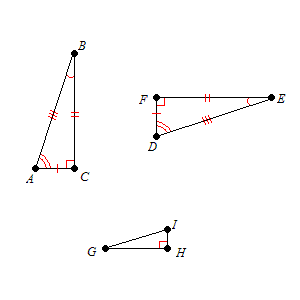# Congruent Triangles

Congruent Triangles are triangles with three pairs of corresponding sides congruent and three pairs of corresponding angles congruent .

In other words, by translating, rotating, and/or flipping one triangle, you can get it to coincide exactly with the other triangle.

If $\Delta ABC$ is congruent to $\Delta DEF$ , then we write

$\Delta ABC\cong \Delta DEF$ .

In the figure below, $\Delta ABC\cong \Delta DEF$ , but $\Delta ABC$ is not congruent to $\Delta GHI$ .Note also that we cannot write $\Delta ABC\cong \Delta DFE$ . The corresponding vertices should come in the right order.